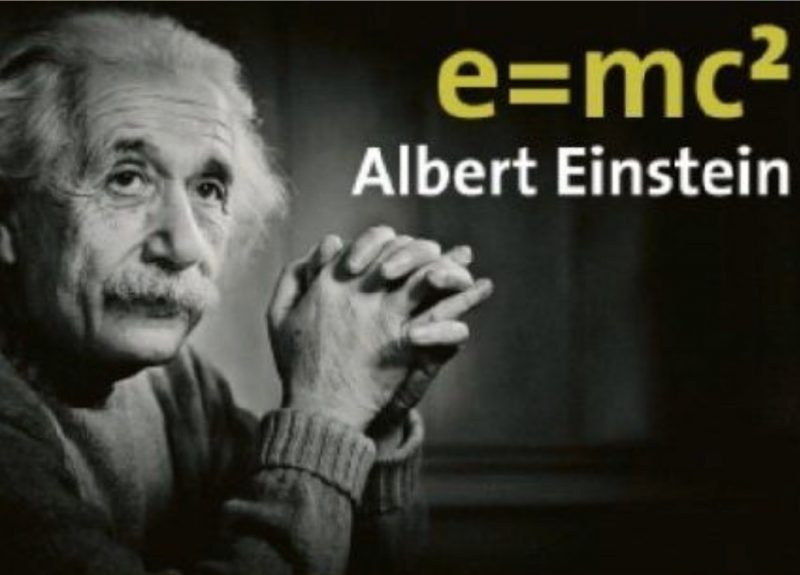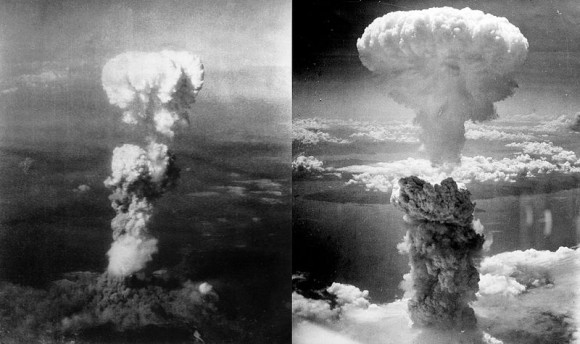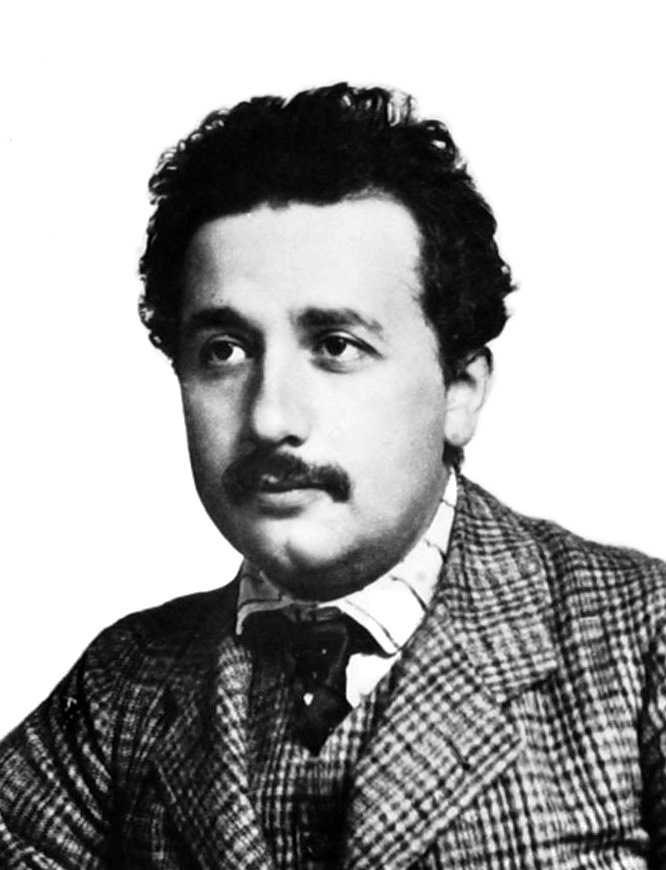Human World

# Einstein’s most famous equation: E=mc2Einstein’s most famous equation, and Einstein himself in his later years. Image via infoastronomy.org.

### Einstein’s most famous equation

In 1905 on tomorrow’s date (September 27) – while employed at a patent office in Bern Switzerland – Albert Einstein published the last of four papers he submitted that year to the journal Annalen der Physik. The first explained the photoelectric effect. The second offered experimental proof of the existence of atoms. And the third introduced the theory of special relativity. Then, in this fourth paper, Einstein explained the relationship between energy and mass, described by E=mc2.

E=mc2 means that, from the standpoint of physics, energy and mass are interchangeable. In the equation:

E is energy
m is mass
c is the speed of light

In other words, energy equals mass times the speed of light squared.

It sounds simple. And its simplicity might obscure the genius, and the innovation of thought, required of Einstein to express it all so elegantly. Mass and energy are interchangeable. Plus, a small amount of mass can equal a large amount of energy. After all, the speed of light is a huge number (186,000 miles per second or 300,000 km/s). And, in Einstein’s famous equation, that huge number is squared. So it doesn’t take much mathematical skill to see that a tiny mass can equal big energy.

E=mc2 explains why the sun and other stars shine. In their interiors, atoms (mass) fuse together, creating the tremendous energy of the sun as described by Einstein’s famous equation.Our sun, as seen with an X-ray telescope, showing the corona, the glowing million-degree plasma that surrounds the sun. The sun’s energy is produced in its interior, via thermonuclear fusion. That is, mass is converted to energy in a way described by Albert Einstein’s famous equation, E=mc2. Image via Yohkoh satellite.

Interestingly, the equation E=mc2 does not appear in Einstein’s fourth paper, which was titled Does the Inertia of a Body Depend Upon Its Energy-Content? That’s because Einstein used V to mean the speed of light in a vacuum and L to mean the energy lost by a body in the form of radiation.

So, in his paper, E=mc2 was not originally written as a formula but as a sentence in German that said (translated into English):

… if a body gives off the energy L in the form of radiation, its mass diminishes by L/V2.Atomic bomb over Hiroshima (left) on August 6, 1945, and Nagasaki (right) on August 9, 1945. Image via Wikimedia Commons.

### Einstein’s most famous equation in bomb-making

This equation enabled scientists to learn how to build a single bomb that could wipe out a city, such as the atomic bombs that destroyed the Japanese cities of Hiroshima and Nagasaki at the end of World War II.

These early atomic bombs worked with nuclear fission, not fusion. But it was the same principle as described by Einstein, that a tiny amount of mass could be converted to a large amount of energy.Albert Einstein, pictured here in the Swiss Patent Office where he did much of his great work. Image via Wikimedia Commons.

### Einstein’s miracle year

Einstein’s 1905 paper describing the interchangeable aspect of mass and energy was one of four papers he published during what’s now called his Annus mirabilis or miracle year.

These four articles forever changed our human perception of mass, energy, space and time. Read more about Einstein’s miracle year.

Bottom line: On September 27, 1905, during his “miracle year,” Albert Einstein published a paper titled Does the Inertia of a Body Depend Upon Its Energy-Content? In the paper, Einstein described the interchangeable nature of mass and energy, or what came to be known as E=mc2.

Posted
September 26, 2021
in
Human World